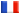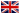# Séminaire de Cryptographie

## Alexander May### RSA problems with Lattice Reduction

This survey addresses the problems of factoring and inverting the RSA function. We define practically relevant relaxed instances of these problems that can be solved in polynomial time. These problem instances are modelled by polynomial equations with small roots. In order to recover the roots, we make use of a method due to Coppersmith which is in turn based on the famous LLL lattice reduction.

As new applications of the method we present an improved Hastad attack on RSA in the case of several RSA encryptions of the same underlying message, and an algorithm for factoring N=pq given 70% of the bits of p in any positions.